# R Weekly Bulletin Vol – IXThis week’s R bulletin will cover topics on how to list files, extracting file names, and creating a folder using R. Hope you like this R weekly bulletin. Enjoy reading!

### Shortcut Keys

1. Run the current chunk - Ctrl+Alt+C
2. Run the next chunk - Ctrl+Alt+N
3. Run the current function definition - Ctrl+Alt+F

### Problem Solving Ideas

#### How to list files with a particular extension

To list files with a particular extension, one can use the pattern argument in the list.files function. For example to list csv files use the following syntax.

Example:

files = list.files(pattern = "\\.csv$") This will list all the csv files present in the current working directory. To list files in any other folder, you need to provide the folder path.  list.files(path = "C:/Users/MyFolder", pattern = "\\.csv$")

$at the end means that this is end of the string. Adding . ensures that you match only files with extension .csv #### Extracting file name using gsub function When we download stock data from google finance, the file’s name corresponds to the stock data symbol. If we want to extract the stock data symbol from the file name, we can do it using the gsub function. The function searches for a match to the pattern argument and replaces all the matches with the replacement value given in the replacement argument. The syntax for the function is given as:  gsub(pattern, replacement, x) where, pattern - is a character string containing a regular expression to be matched in the given character vector. replacement - a replacement for matched pattern. x - is a character vector where matches are sought. In the example given below, we extract the file name for files stored in the “Reading MFs” folder. We have downloaded the stock price data in R working directory for two companies namely, MRF and PAGEIND Ltd. Example: folderpath = paste(getwd(), "/Reading MFs", sep = "") temp = list.files(folderpath, pattern = "*.csv") print(temp)  "MRF.csv" "PAGEIND.csv" gsub("*.csv$", "", temp)

 "MRF"   "PAGEIND"

#### Create a folder using R

One can create a folder via R with the help of the “dir.create” function. The function creates a folder with the name as specified in the last element of the path. Trailing path separators are discarded.

The syntax is given as:

dir.create(path, showWarnings = FALSE, recursive = FALSE)

Example:

dir.create("D:/RCodes", showWarnings = FALSE, recursive = FALSE)

This will create a folder called “RCodes” in the D drive.

### Functions Demystified

#### select function

The select function comes from the dplyr package and can be used to select certain columns of a data frame which you need. Consider the data frame “df” given in the example.

Example:

library(dplyr)
Ticker = c("INFY", "TCS", "HCL", "TECHM")
OpenPrice = c(2012, 2300, 900, 520)
ClosePrice = c(2021, 2294, 910, 524)
df = data.frame(Ticker, OpenPrice, ClosePrice)
print(df)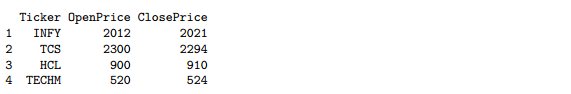# Suppose we wanted to select the first 2 columns only. We can use the names of the columns in the
# second argument to select them from the main data frame.

subset_df = select(df, Ticker:OpenPrice)
print(subset_df)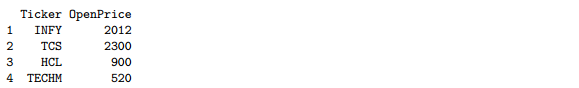# Suppose we want to omit the OpenPrice column using the select function. We can do so by using
# the negative sign along with the column name as the second argument to the function.

subset_df = select(df, -OpenPrice)
print(subset_df)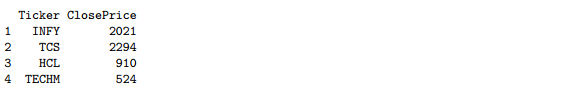# We can also use the 'starts_with' and the 'ends_with' arguments for selecting columns from the
# data frame. The example below will select all the columns which end with the word 'Price'.

subset_df = select(df, ends_with("Price"))
print(subset_df)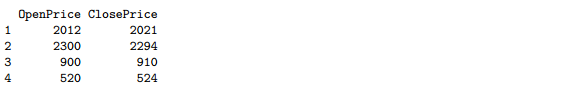#### filter function

The filter function comes from the dplyr package and is used to extract subsets of rows from a data frame. This function is similar to the subset function in R.

Example:

library(dplyr)
Ticker = c("INFY", "TCS", "HCL", "TECHM")
OpenPrice = c(2012, 2300, 900, 520)
ClosePrice = c(2021, 2294, 910, 524)
df = data.frame(Ticker, OpenPrice, ClosePrice)
print(df)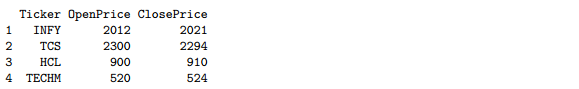# Suppose we want to select stocks with closing prices above 750, we can do so using the filter
# function in the following manner:

subset_df = filter(df, ClosePrice > 750)
print(subset_df)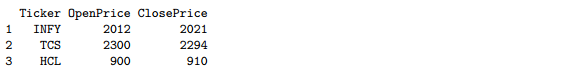# One can also use a combination of conditions as the second argument in filtering a data set.

subset_df = filter(df, ClosePrice > 750 & OpenPrice < 2000)
print(subset_df)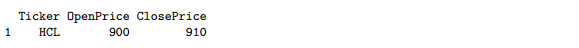#### arrange function

The arrange function is part of the dplyr package, and is used to reorder rows of a data frame according to one of the columns. Columns can be arranged in descending order or ascending order by using the special desc() operator.

Example:

library(dplyr)
Ticker = c("INFY", "TCS", "HCL", "TECHM")
OpenPrice = c(2012, 2300, 900, 520)
ClosePrice = c(2021, 2294, 910, 524)
df = data.frame(Ticker, OpenPrice, ClosePrice)
print(df)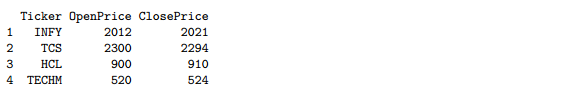# Arrange in descending order

subset_df = arrange(df, desc(OpenPrice))
print(subset_df)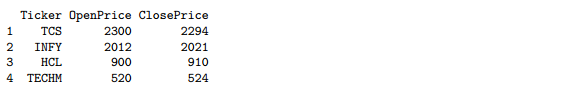# Arrange in ascending order.

subset_df = arrange(df, -desc(OpenPrice))
print(subset_df)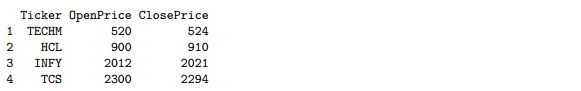### Next Step

We hope you liked this bulletin. In the next weekly bulletin, we will list more interesting ways and methods plus R functions for our readers.

Update

We have noticed that some users are facing challenges while downloading the market data from Yahoo and Google Finance platforms. In case you are looking for an alternative source for market data, you can use Quandl for the same.